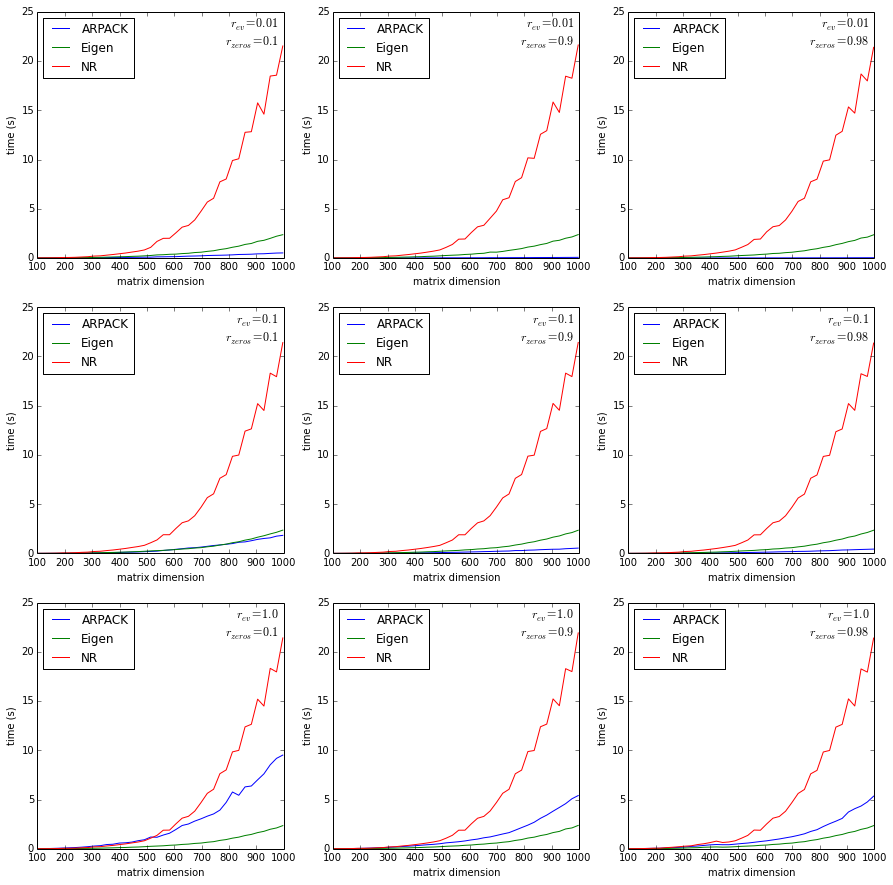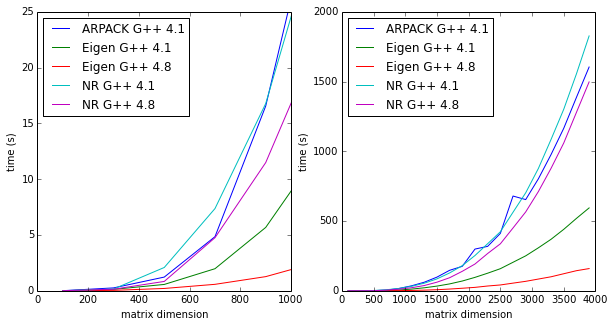# Performance of ARPACK, Eigen, and Numerical Recipes¶

Burkhard Ritter, Christopher Polachic, February 2014

We compare the performance of the Numerical Recipes (C++ version, 3rd edition), ARPACK and Eigen eigensolvers for different real symmetric matrices with varying sparseness. Both Numerical Recipes and Eigen are dense eigensolvers and always compute the full eigenvalue spectrum, whereas ARPACK uses sparse matrices and is typically used to calculate only a few eigenvalues and not the full spectrum.

In :
def create_data_files():
i_d = 0
for r_ev in [0.01,0.1,1.0]:
for r_zeros in [0.1,0.9,0.98]:
i_d += 1
fn = 'performance_nr.{}.dat'.format(i_d)
!./build/arpaca_performance_plot_nr 100 1000 40 10 $r_ev$r_zeros > $fn  In : # Takes a very long time # create_data_files()  In : import re def read_data_file(filename): f = open(filename) s = f.read() f.close() n_req = None r_ev = None r_zeros = None d = None m = re.search(r'n_rep=(.+)$',s,re.MULTILINE)
if m:
n_rep = int(m.group(1))
m = re.search(r'r_ev=(.+)$',s,re.MULTILINE) if m: r_ev = float(m.group(1)) m = re.search(r'r_zeros=(.+)$',s,re.MULTILINE)
if m:
r_zeros = float(m.group(1))
return (n_rep,r_ev,r_zeros,d)

def subplot_data_file(p, filename):
p.plot(d[:,0],d[:,1],label='ARPACK')
p.plot(d[:,0],d[:,2],label='Eigen')
p.plot(d[:,0],d[:,3],label='NR')
p.set_xlabel('matrix dimension')
p.set_ylabel('time (s)')
p.legend(loc='upper left')
#p.set_xscale('log')
#p.set_yscale('log')
#if r_ev == 1.0:
#    p.set_ylim((0,300))
p.text(0.98,0.98,
'$r_{{ev}} = {}$\n$r_{{zeros}} = {}$'.format(r_ev,r_zeros),
fontsize='12',
verticalalignment='top',
horizontalalignment='right',
transform=p.transAxes)

In :
%matplotlib inline
import matplotlib.gridspec as gridspec

rows = 3
cols = 3

fig = plt.figure(figsize=(cols*5,rows*5))
#gs = gridspec.GridSpec(rows,cols)
i_p = 0

for i in range(1,10):
i_p += 1
subplot_data_file(p, 'performance_nr.{}.dat'.format(i))The time the eigensolvers take to diagonalize a random matrix of a given size. Here, $r_{ev}$ is the "ratio" of eigenvalues. Hence $r_{ev} = 0.1$ means calculating 10% of the eigenvalues, for ARPACK only. Eigen and Numerical Recipes always calculate all eigenvalues. $r_{zeros}$ is the "ratio" of zeros. Hence $r_{zeros} = 0.25$ means 25% sparse. Both Eigen and Numerical Recipes are not affected by $r_{ev}$ and $r_{zeros}$ and thus the corresponding curves should be the same for all plots above.

This test was run on OS X 10.9.1, the compiler was GNU G++ 4.8.2 (installed from MacPorts). Arpack is 3.1.4 (it's Arpack-ng), installed from MacPorts. Arpaca was used as the Arpack wrapper.

In :
def subplot_chris(p,xlim=None,ylim=None):
# columns are: N  NRg++4.1.2 Arpack4.1.2 Eigen4.1.2 NR4.8.2 Eigen4.8.2
filename = 'performance_chris.dat'
p.plot(d[:,0],d[:,2],label='ARPACK G++ 4.1')
p.plot(d[:,0],d[:,3],label='Eigen G++ 4.1')
p.plot(d[:,0],d[:,5],label='Eigen G++ 4.8')
p.plot(d[:,0],d[:,1],label='NR G++ 4.1')
p.plot(d[:,0],d[:,4],label='NR G++ 4.8')
p.set_xlabel('matrix dimension')
p.set_ylabel('time (s)')
p.legend(loc='upper left')
if xlim: p.set_xlim(xlim)
if ylim: p.set_ylim(ylim)

rows = 1
cols = 2

fig = plt.figure(figsize=(cols*5,rows*5))
i_p = 0

i_p += 1
subplot_chris(p,(0,1000),(0,25))

i_p += 1
subplot_chris(p)A very similar test, this time run on WestGrid's Jasper (Linux). Uses latest Arpack from Rice university and Arpack++ as the wrapper. The matrix is the Hamiltonian from a real physical problem, it's about 1% to 2% sparse. Numerical Recipes and Eigen are compiled with two different versions of the GNU G++ compiler, as indicated. The full spectrum of eigenvalues is computed. This scenario corresponds to the $r_{ev} = 1.0$ and $r_{zeros} = 0.98$ (the bottom right-most) plot in the last graph.

While the general trends agree, there are some notable differences: Arpack is much slower here than above. Reversely, Numerical Recipes is faster in this test, compared to the last.

It should be noted that ARPACK was used in a black box like fashion (via the wrappers) and there might be considerable room for improving its performance, for example by compromising on precision (we use machine precision) or just using it in a cleverer way. To compute the full spectrum we actually run ARPACK twice, once to get all but one eigenvalue, starting from the smallest, and once the compute the missing largest eigenvalue. (ARPACK does not seem to allow to compute the whole eigenvalue spectrum directly, at least not in the simple direct manner we are using via the wrappers.)

We summarize the results of these tests:

1. Never ever use Numerical Recipes, it's extremely slow.
2. Use new compilers. They are significantly faster than the old ones, especially for sophisticated C++ libraries like Eigen.
3. To calculate only a couple of eigenvalues, use Arpack; and it's increasingly faster for increasingly sparse matrices. No surprises here.
4. To calculate all eigenvalues, use Eigen.